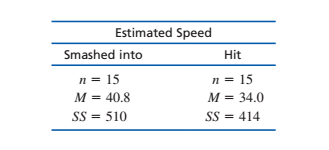# Estimated SpeedSmashed intoHitn = 15n = 15M = 40.8M = 34.0%3DSS = 414SS = 510%3!

Question
1 views

10. In 1974, Loftus and Palmer conducted a classic study demonstrating how the language used to ask a question can influence eyewitness memory. In the study, college students watched a film of an automobile accident and then were asked questions about what they saw. One group was asked, “About how fast were the cars going when they smashed into each other?” Another group was asked the same question except the verb was changed to “hit” instead of “smashed into.” The “smashed into” group reported significantly higher estimates of speed than the “hit” group. Suppose a researcher repeats this study with a sample of today’s college students and obtains the following results.

a. Do the results indicate a significantly higher estimated speed for the “smashed into” group? Use a one-tailed test with α = .01.

b. Compute the estimated value for Cohen’s d to measure the size of the effect.

c. Write a sentence demonstrating how the results of the hypothesis test and the measure of effect size would appear in a research report.help_outlineImage TranscriptioncloseEstimated Speed Smashed into Hit n = 15 n = 15 M = 40.8 M = 34.0 %3D SS = 414 SS = 510 %3! fullscreen
check_circle

Step 1

Introduction:

The null and alternative hypotheses are:

H0: The estimated speeds for the “smashed into” and “hit” groups are not different.

H1: The estimated speed for the “smashed into” group is greater than that of the “hit” group.

Step 2

Formulae used:

Denote SS as the sum of squares, Sp2 as the pooled variance, Se as the standard error of estimate.

Step 3

Calculation (a):

From the formula for the pooled variance, it can be understood that SS1 = (n1 – 1) S12, SS2 = (n2 – 1) S22.

Pooled variance:

Sp2

= (S12 + S22) / (n1 + n2 – 2)

= (510 + 414) / (15 + 15 – 2)

= 924/28

= 33.

Standard error:

Se

= Sp / √ (1/n1 + 1/n2)

= √ [Sp2 / (1/n1 + 1/n2)]

= √ [33/(1/15 + 1/15)]

≈ 2.0976.

Test statistic:

t

= (1 – 2)/Se

= (40.8 – 34.0)/2.0976

≈ 3.2418.

Conclusion:

The degrees of freedom for the t-test is n1 + n2 – 2 = 28.

For the right-tailed test at level of significance 0.01 with 28 degrees of freedom, the t-test cutoff value is 2.47.

Decision rule: Reject H...

### Want to see the full answer?

See Solution

#### Want to see this answer and more?

Solutions are written by subject experts who are available 24/7. Questions are typically answered within 1 hour.*

See Solution
*Response times may vary by subject and question.
Tagged in

### Statistics